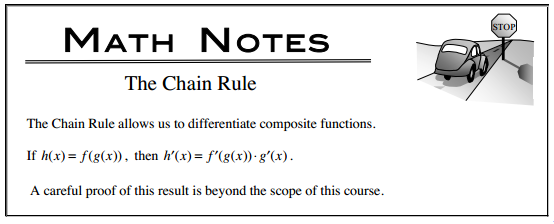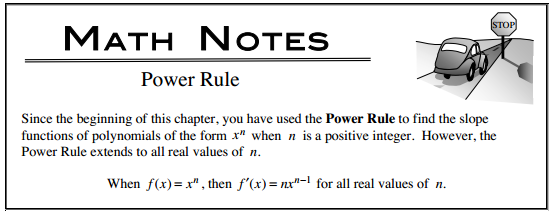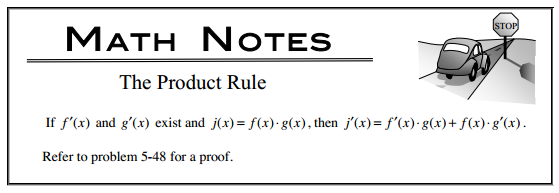### Home > CALC > Chapter 5 > Lesson 5.2.2 > Problem5-75

5-75.

Use your derivative tools to find $\frac { d h } { d x }$.

1. $h(x) =\operatorname{cos}(x^2)$

1. $h ( x ) = \sqrt [ 3 ] { \operatorname { sin } x }$

1. $h(x) =6x^{2/3}$

1. $h ( x ) = \frac { 1 } { 2 } x ^ { 5 } \sqrt { x - 1 }$

1. $h ( x ) = 2 \operatorname { sin } ( \frac { 1 } { x } )$

1. $h(x) = −4\operatorname{ cos }x\operatorname{ sin }x$